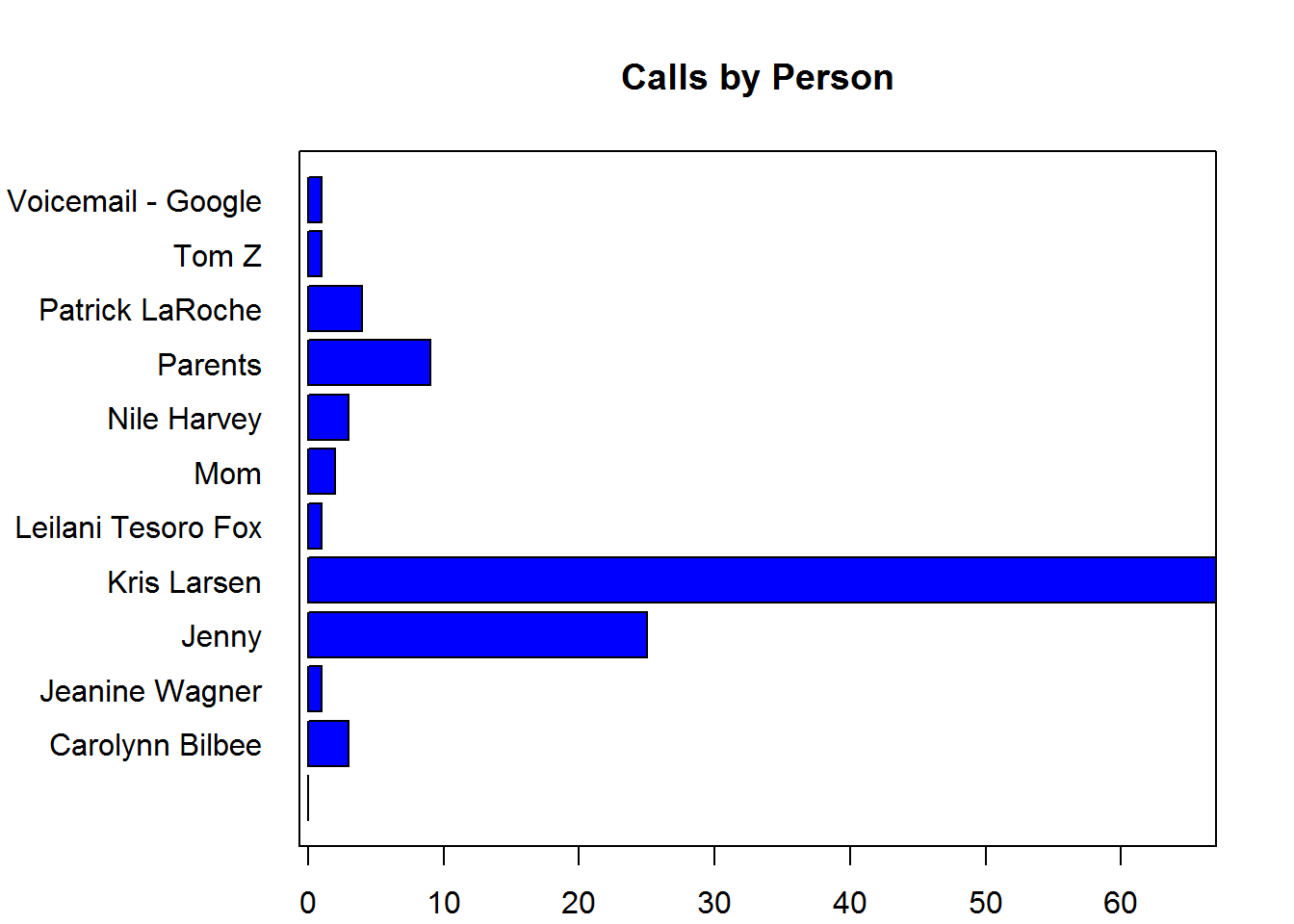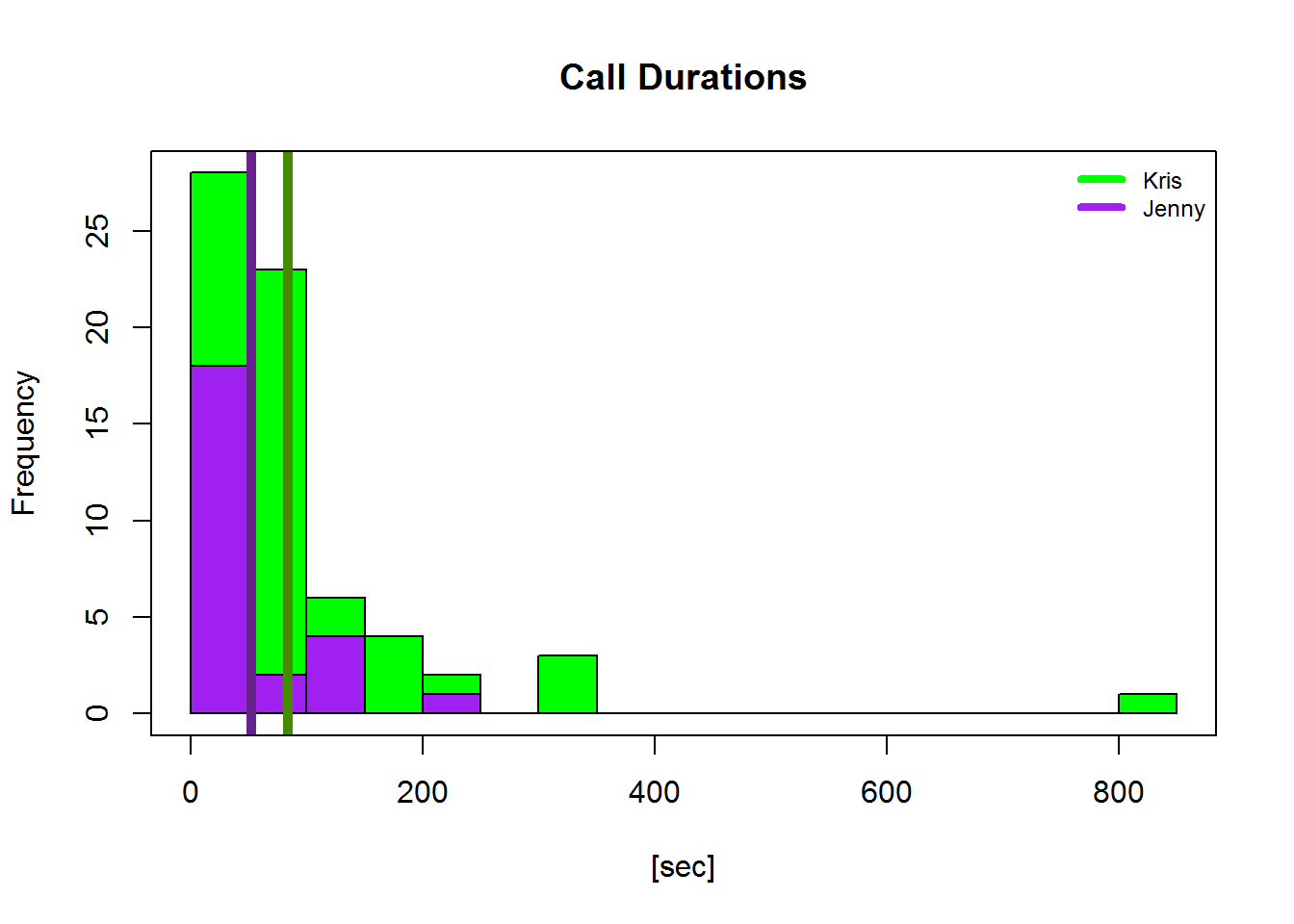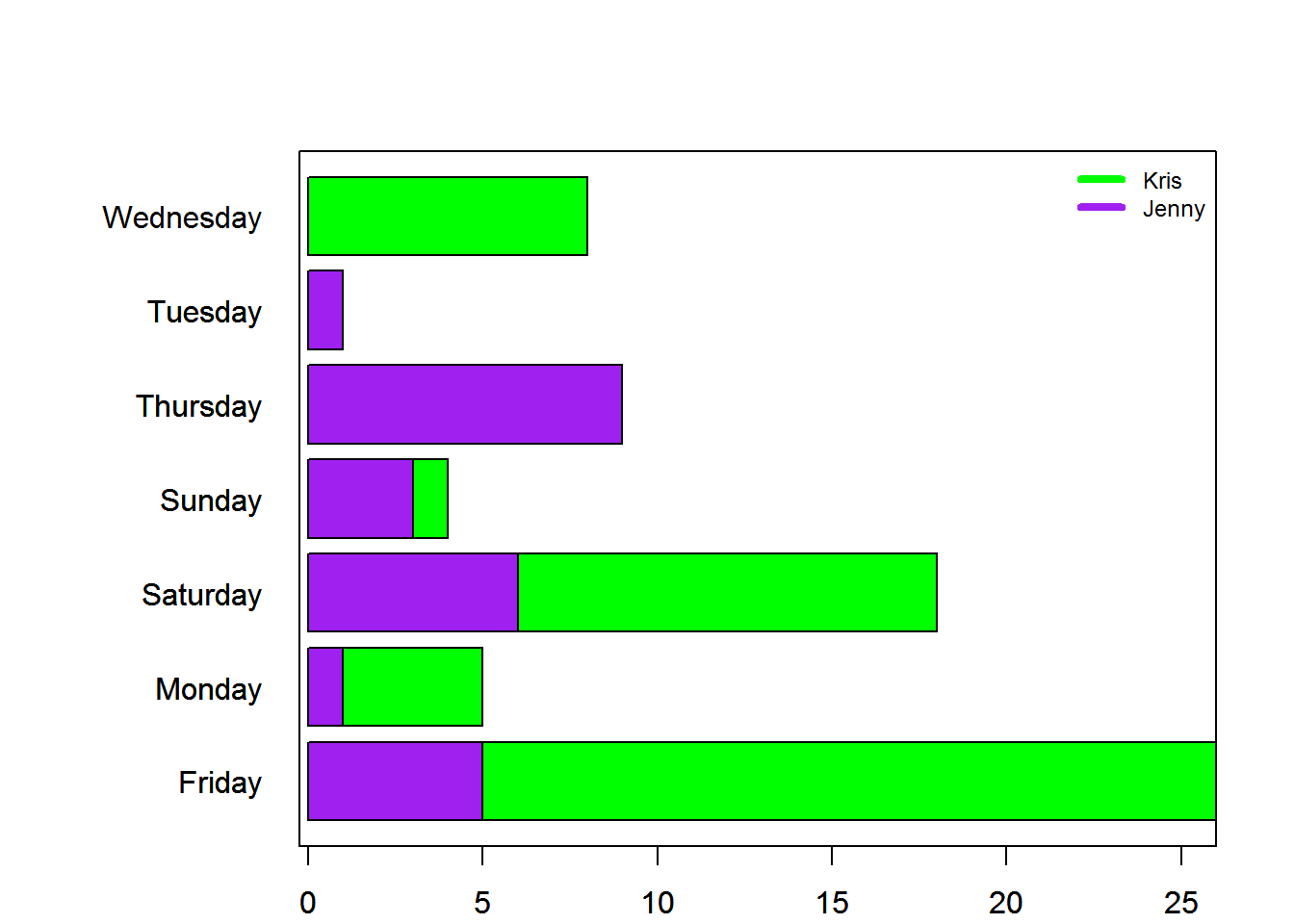## Who calls me more: Jen or Kris?

Time to answer this important question: does my wife call me more or Kris? To answer, I logged all incoming calls for 18 months, using this recipe from IFTTT.

First, I downloaded the .csv file, and added a header and slightly modified the date stamp column. Two subsets are created: one for Kris’ calls, and one for Jenny’s. Both subsets have a date object column created by concatenating monthh,day, and year factors previously read in.

setwd("C:/Users/Jeffrey.Waring/R projects/Call logging")

kris <- df[df$Caller=="Kris Larsen",] #Now create a date column kris$Date<-as.Date(paste(as.character(kris$Month),as.character(kris$Day),' ',as.character(kris$Year)),format="%B %d,%Y") #and a weekday column kris$Weekday<-c("Sunday", "Monday", "Tuesday", "Wednesday", "Thursday",
"Friday", "Saturday")[as.POSIXlt(kris$Date)$wday + 1]

jenny <- df[df$Caller=="Jenny",] #Now create a date column jenny$Date<-as.Date(paste(as.character(jenny$Month),as.character(jenny$Day),' ',as.character(jenny$Year)),format="%B %d,%Y") #and a weekday column jenny$Weekday<-c("Sunday", "Monday", "Tuesday", "Wednesday", "Thursday",
"Friday", "Saturday")[as.POSIXlt(jenny$Date)$wday + 1]

In total, I recieved: 192 calls from the dates:

##  "2014-05-22" "2015-11-20"

Of these, 75 calls

(or 39.1%) were from unknown numbers

These unknown numbers are excluded from further analysis.

## Now, some analysis

Clearly Kris is the winner for total calls:

par(mar = c(2, 8, 4, 2) + 0.1, mgp = c(4, 1, 0), las = 2)
barplot(table(df2$Caller),horiz=TRUE,col="blue",main="Calls by Person",xlab="# of calls",las=1) box()With a given average calltime of 83.6 seconds, Kris’ calls are also longer than average (77.3 seconds) and Jens’ (52.4 seconds): hist(kris$length,col="green",breaks = 20,main="Call Durations",xlab="[sec]")
hist(jenny$length,col="purple",add=TRUE,breaks=5) abline(v=mean(kris$length),col="chartreuse4",lwd=5)
abline(v=mean(jenny$length),col="darkorchid4",lwd=5) legend('topright', c("Kris","Jenny"),lty=1, lwd=4,bty='n', cex=.75,col=c("green","purple")) box()Finally, and perhaps least surprisingly, Kris’ calls tend to skew to the weekends: when plans need to be made. Jens’ calls tend to be more evenly distributed, with the exception of Wednesday. par(mar = c(2, 8, 4, 2) + 0.1, mgp = c(4, 1, 0), las = 2) barplot(table(kris$Weekday),las=1,hori=TRUE,col="green")
box()